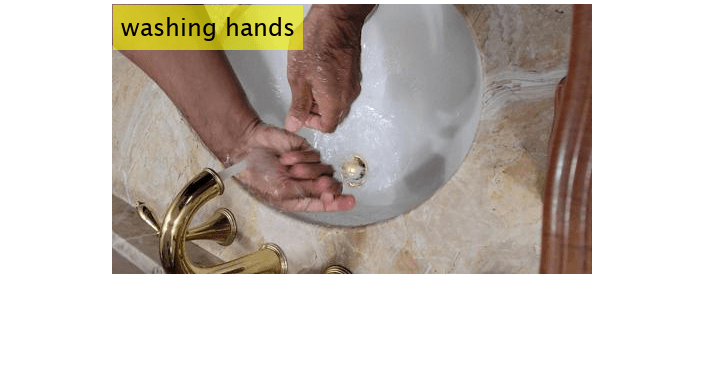# forward

Compute video classifier outputs for training

Since R2021b

## Syntax

``dLYVideo = forward(i3d,dlXVideo)``
``[dLYVideo,stateVideo] = forward(i3d,dlXVideo)``
``[dLYVideo,dlYFlow] = forward(i3d,dlXVideo,dlXFlow)``
``[dLYVideo,dlYFlow,stateVideo,stateFlow] = forward(i3d,dlXVideo,dlXFlow)``

## Description

example

````dLYVideo = forward(i3d,dlXVideo)` computes the video classifier outputs for training. You can use this function with `dlfeval` (Deep Learning Toolbox) to automatically compute gradients for updating the learnable parameters of the video classifier. `i3d` is specified as an `inflated3dVideoClassifier` classifier object. Use this syntax when you set the `OpticalFlowMethod` property of the classifier object to `"none"`.```
````[dLYVideo,stateVideo] = forward(i3d,dlXVideo)` also returns the updated video network state. The output, `stateVideo`, contains information maintained by the classifier between training iterations. For example, the state of batch normalization operation.```
````[dLYVideo,dlYFlow] = forward(i3d,dlXVideo,dlXFlow)` also returns the optical flow outputs from the classifier for training. Use this syntax when you set the `OpticalFlowMethod` property of the classifier object to `"Farneback"`. ```
````[dLYVideo,dlYFlow,stateVideo,stateFlow] = forward(i3d,dlXVideo,dlXFlow)` also returns the updated video network and the optical flow network states.```

## Examples

collapse all

This example shows how to compute video classifier outputs for training. To learn more about how to train a video classifier network for your dataset, see Gesture Recognition using Videos and Deep Learning.

Load a video classifier pretrained on the Kinetics-400 data set.

`sf = slowFastVideoClassifier;`

Specify the video file name.

`videoFilename = "washingHands.avi";`

Create a `VideoReader` to read the video frames.

`reader = VideoReader(videoFilename);`

Read the required number of video frames corresponding to the video classifier network from the beginning of the video file. The required number of frames is defined by the value of the 4th element of the `InputSize` property of the video classifier.

```sequenceLength = sf.InputSize(4); sequenceRange = [1,sequenceLength]; videoFrames = read(reader,sequenceRange);```

Resize the video frames for training. The required height and width are defined by the first two elements of the `InputSize` property of the video classifier.

```heightWidth = sf.InputSize(1:2); resized = imresize(videoFrames,heightWidth);```

Convert the input to type `single`.

`resized = single(resized);`

Rescale the input between 0 and 1.

```minValue = sf.InputNormalizationStatistics.Min; maxValue = sf.InputNormalizationStatistics.Max; minValue = reshape(minValue,1,1,3); maxValue = reshape(maxValue,1,1,3); resized = rescale(resized,0,1,InputMin=minValue,InputMax=maxValue);```

Normalize the video data using the mean and standard deviation.

```meanValue = sf.InputNormalizationStatistics.Mean; stdValue = sf.InputNormalizationStatistics.StandardDeviation; meanValue = reshape(meanValue,1,1,3); stdValue = reshape(stdValue,1,1,3); resized = resized - meanValue; resized = resized./stdValue;```

Convert the input to a `dlarray` object.

```dlVideo = dlarray(resized,"SSCTB"); trainingActivations = forward(sf,dlVideo);```

Find the class label corresponding to the maximum score.

```[score,idx] = max(trainingActivations); label = sf.Classes(idx)```
```label = categorical washing hands ```
`score`
```score = 1(S) × 1(S) × 1(S) × 1(C) × 1(B) single dlarray 0.0026 ```

Display the class label.

```frame = videoFrames(:,:,:,end); frame = insertText(frame,[2,2],string(label),FontSize=24); imshow(frame)```## Input Arguments

collapse all

Classifier, specified as an `inflated3dVideoClassifier` object.

Video input, specified as an H-by-W-by-C-by-T-by-B `SSCTB` formatted `dlarray` (Deep Learning Toolbox) object that corresponds to the video input of the classifier.

• H — Height.

• W — Width.

• C — Number of channels. The number of channels must be equal to the channels value of the `InputSize` property of the classifier object.

• T — Number of frames. The number of frames must be equal to the frames value of the `InputSize` property of the classifier object.

• B — Batch size.

Video and optical flow input, specified as an H-by-W-by-C-by-T-by-B `SSCTB` formatted `dlarray` (Deep Learning Toolbox) object that corresponds to the video and optical flow input of the classifier.

• H — Height.

• W — Width.

• C — Number of channels. The number of channels must be equal to the channels value of the `InputSize` property of the classifier object.

• T — Number of frames. The number of frames must be equal to the frames value of the `InputSize` property of the classifier object.

• B — Batch size.

## Output Arguments

collapse all

Activations of the video network, returned as a formatted `dlarray` (Deep Learning Toolbox) object .

Updated video network state, returned as a table with three columns:

• `Layer` — Layer name, specified as a string scalar.

• `Parameter` — Parameter name, specified as a string scalar.

• `Value` — Value of the parameter, specified as a `dlarray` object.

The network state contains information remembered by the network between iterations. For example, the state of the LSTM and batch normalization layers.

During training or inference, you can update the network state using the output of the `forward` and `predict` functions.

Activations of the optical flow network, returned as a formatted `dlarray` (Deep Learning Toolbox) object .

Updated optical flow network state, returned as a table with three columns:

• `Layer` — Layer name, specified as a string scalar.

• `Parameter` — Parameter name, specified as a string scalar.

• `Value` — Value of the parameter, specified as a `dlarray` object.

The network state contains information remembered by the network between iterations. For example, the state of LSTM and batch normalization layers.

During training or inference, you can update the network state using the output of the `forward` and `predict` functions.

## Version History

Introduced in R2021b